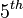# SmallGroup(32,5)

View a complete list of particular groups (this is a very huge list!)[SHOW MORE]

## Position in classifications

Type of classification Position/number in classification
GAP ID$(32,5)$, i.e.,$5^{th}$ among groups of order 32
Hall-Senior number 20 among groups of order 32
Hall-Senior symbol$32\Gamma_2j_1$

## Arithmetic functions

Want to compare and contrast arithmetic function values with other groups of the same order? Check out groups of order 32#Arithmetic functions

## Group properties

Property Satisfied? Explanation Comment
group of prime power order Yes
nilpotent group Yes prime power order implies nilpotent
supersolvable group Yes via nilpotent: finite nilpotent implies supersolvable
solvable group Yes via nilpotent: nilpotent implies solvable
abelian group No

## GAP implementation

### Group ID

This finite group has order 32 and has ID 5 among the groups of order 32 in GAP's SmallGroup library. For context, there are 51 groups of order 32. It can thus be defined using GAP's SmallGroup function as:

SmallGroup(32,5)

For instance, we can use the following assignment in GAP to create the group and name it$G$:

gap> G := SmallGroup(32,5);

Conversely, to check whether a given group$G$ is in fact the group we want, we can use GAP's IdGroup function:

IdGroup(G) = [32,5]

or just do:

IdGroup(G)

to have GAP output the group ID, that we can then compare to what we want.